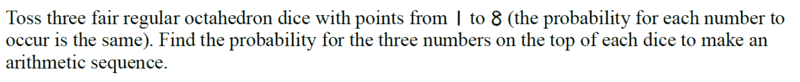# Probability of getting arithmetic sequence from 3 octahedron dice

songoku
Homework Statement:
Relevant Equations:
Probability

Arithmetic SequenceI try to list all the possible sequences:
1 2 3
1 3 5
1 4 7
2 3 4
2 4 6
2 5 8
3 4 5
3 5 7
4 5 6
4 6 8
5 6 7
6 7 8

I get 12 possible outcomes, so the probability is ##\frac{12 \times 3!}{8^3}=\frac{9}{64}##

But the answer key is ##\frac{5}{32}## . Where is my mistake? Thanks

Homework Helper
Gold Member
2022 Award
Relevant Equations:: Probability

Arithmetic Sequence

Where is my mistake?
In the sequence a, a+b, a+2b, what are all the possible values of b?

•songoku
songoku
In the sequence a, a+b, a+2b, what are all the possible values of b?
I think for this case the difference should be positive integer so b can be 1, 2, or 3

Homework Helper
Gold Member
2022 Award
I think for this case the difference should be positive integer so b can be 1, 2, or 3
I disagree. 1, 1, 1 is a perfectly good arithmetic sequence.

••songoku and Delta2
Homework Helper
Gold Member
I know I ll spoil abit the solution but in order to be a bit more formal and since the dice is 8-ply it will have to be $$1\leq a+2b\leq8\Rightarrow \frac{1-a}{2}\leq b\leq \frac{8-a}{2}$$ and ofc $$b\geq 0$$.

•songoku
Homework Helper
Gold Member
Anyway I think you did find the b correctly, except you didn't took the case b=0 (and tbh I myself didn't think of that). If you add the 8 cases (a,a,a) ,a=1...8 to your result, you get the answer key.

•songoku
songoku
I disagree. 1, 1, 1 is a perfectly good arithmetic sequence.
I know I ll spoil abit the solution but in order to be a bit more formal and since the dice is 8-ply it will have to be $$1\leq a+2b\leq8\Rightarrow \frac{1-a}{2}\leq b\leq \frac{8-a}{2}$$ and ofc $$b\geq 0$$.
Is 1, 1, 1 can also be called geometric sequence?

Homework Helper
Gold Member
Is 1, 1, 1 can also be called geometric sequence?
Yes it is arithmetic sequence with ##\omega=0## and geometric sequence with ##\omega=1##.

•songoku
songoku
Yes it is arithmetic sequence with ##\omega=0## and geometric sequence with ##\omega=1##.
How about 0, 0, 0? Can that also be called both arithmetic and geometric sequence?

Homework Helper
Gold Member
I think yes but why are you asking these questions...

•songoku
Homework Helper
Gold Member
0 isn't a possible number from the dice.

•songoku
songoku
I think yes but why are you asking these questions...
I just want to know so if I do other questions I know which one I can consider as arithmetic or geometric sequence.

Thank you very much for the help and explanation haruspex and Delta2

•Delta2